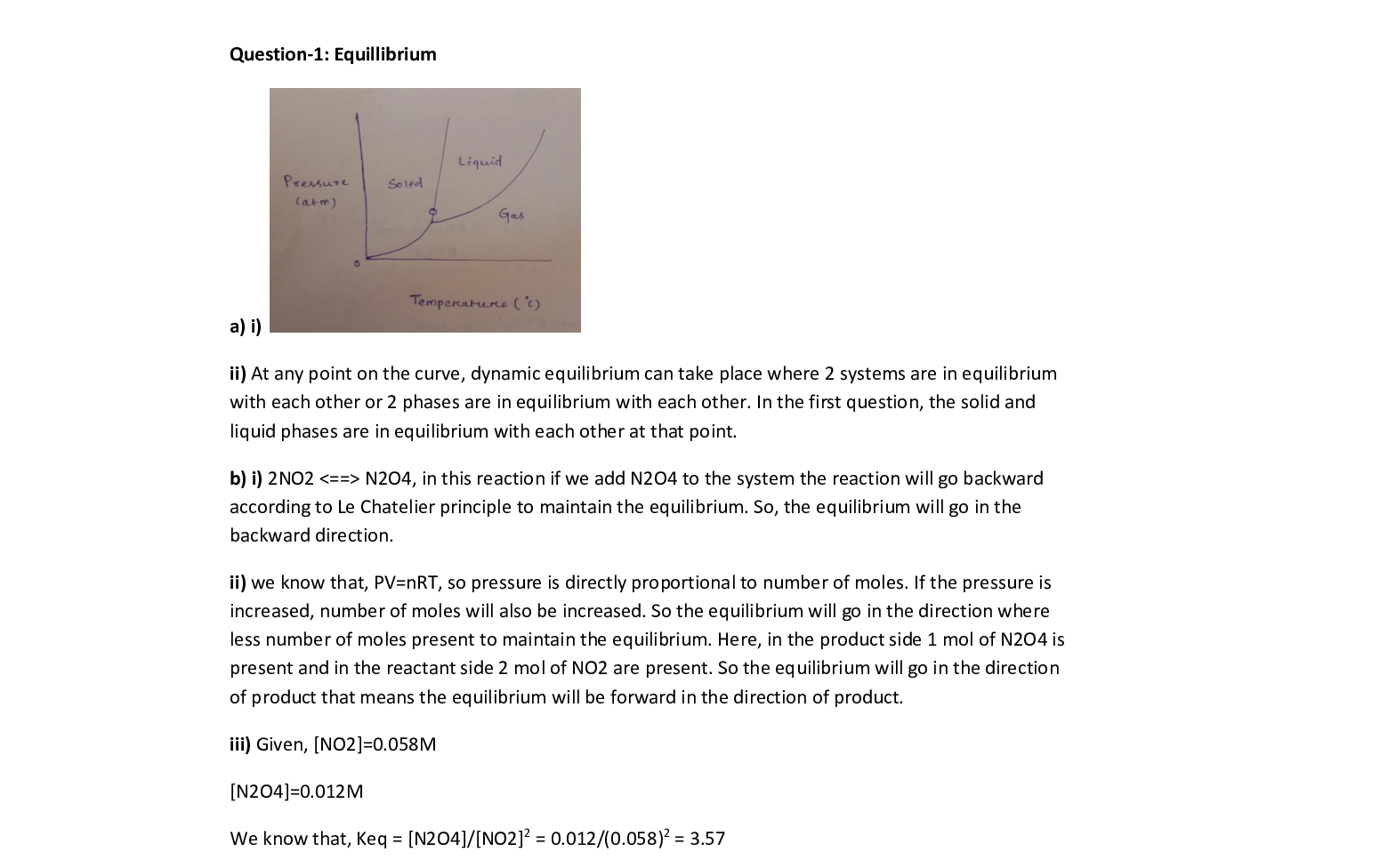Question

Organic Chemistry

a. Use the CO, phase diagram to answer the following questions. (4 points)

i. Circle any point on the CO2 phase diagram that represents a system at dynamicequilibrium. (2 points)

ii. What is happening at that point that makes it a dynamic equilibrium? (2 points)

\text { Use the reaction } 2 \mathrm{NO}_{2}(g) \rightleftharpoons \mathrm{N}_{2} \mathrm{O}_{4}(g) \text { to answer the following questions. (6 points) }

i. What does Le Châtelier's principle predict would happen to the equilibrium conditions ifN,04 were added to the system? (2 points)

ii. What would happen to the equilibrium if the pressure were increased? (2 points)Verified### Question 45767Organic Chemistry

5. The iodine for this experiment is in the form of i3, and not l2
Why is this form of iodine used?

### Question 45766Organic Chemistry

What happened to the hydrogen atoms that were present in the ascorbic acıd?а.
b. Acid was added in the titration, but it is not present in equation (2) in a stoichiometric amount.So, what is the purpose of the acid?

### Question 45765Organic Chemistry

3. Write the net ionic equation for the conversion of iodine, I, to iodide, I. What kind of reaction is this?

### Question 45764Organic Chemistry

2. Vitamin C is ascorbic acid. If you examine the structure of ascorbic acid given in the Background section (equation 2), there is no -COOH group present. In the structure shown, the ascorbic acid isas a lactone or cyclic ester. Write the equation for the hydrolysis (cleavage by addition of water) of the lactone to the carboxylic acid.

### Question 45763Organic Chemistry

1. Use the average concentration of vitamin C from your data, and calculate the volume of fruit drink that would satisfy your minimum daily requirement of vitamin C.

### Question 45762Organic Chemistry

. To mark the endpoint of the titration, what indicator is used and how does it work?

### Question 45761Organic Chemistry

In the reaction under study, what is the molar ratio between the abscorbic acid and the iodine?

### Question 45760Organic Chemistry

What enzyme is kept in its active form by the presence of vitamin C in the diet?

### Question 45759Organic Chemistry

5. What disease does this vitamin prevent?

### Question 45758Organic Chemistry

4. How does vitamin C behave in a biological system?

### Submit query